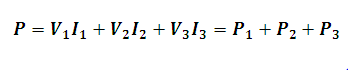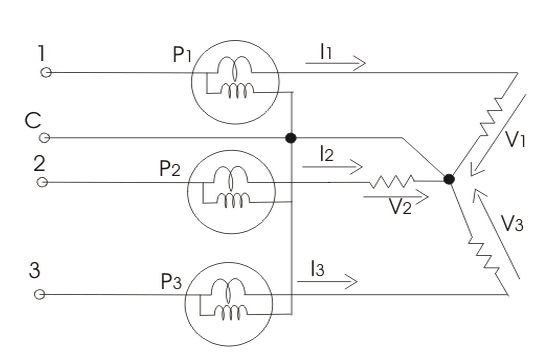# Three Phase Power Using Three Watt-meters Calculator

This Calctown Calculator calculates the three phase power using three watt-meter method.

kW
kW
kW

#### Result

kWwhere

P1 = reading of watt-meter 1

P2 = reading of watt-meter 2

P3 = reading of watt-meter 3

V1 = voltage difference between 1 and c

V2 = voltage difference between 2 and c

V3 = voltage difference between 3 and c

I1 = current through watt-meter 1

I2 = current through watt-meter 2

I3 = current through watt-meter 3